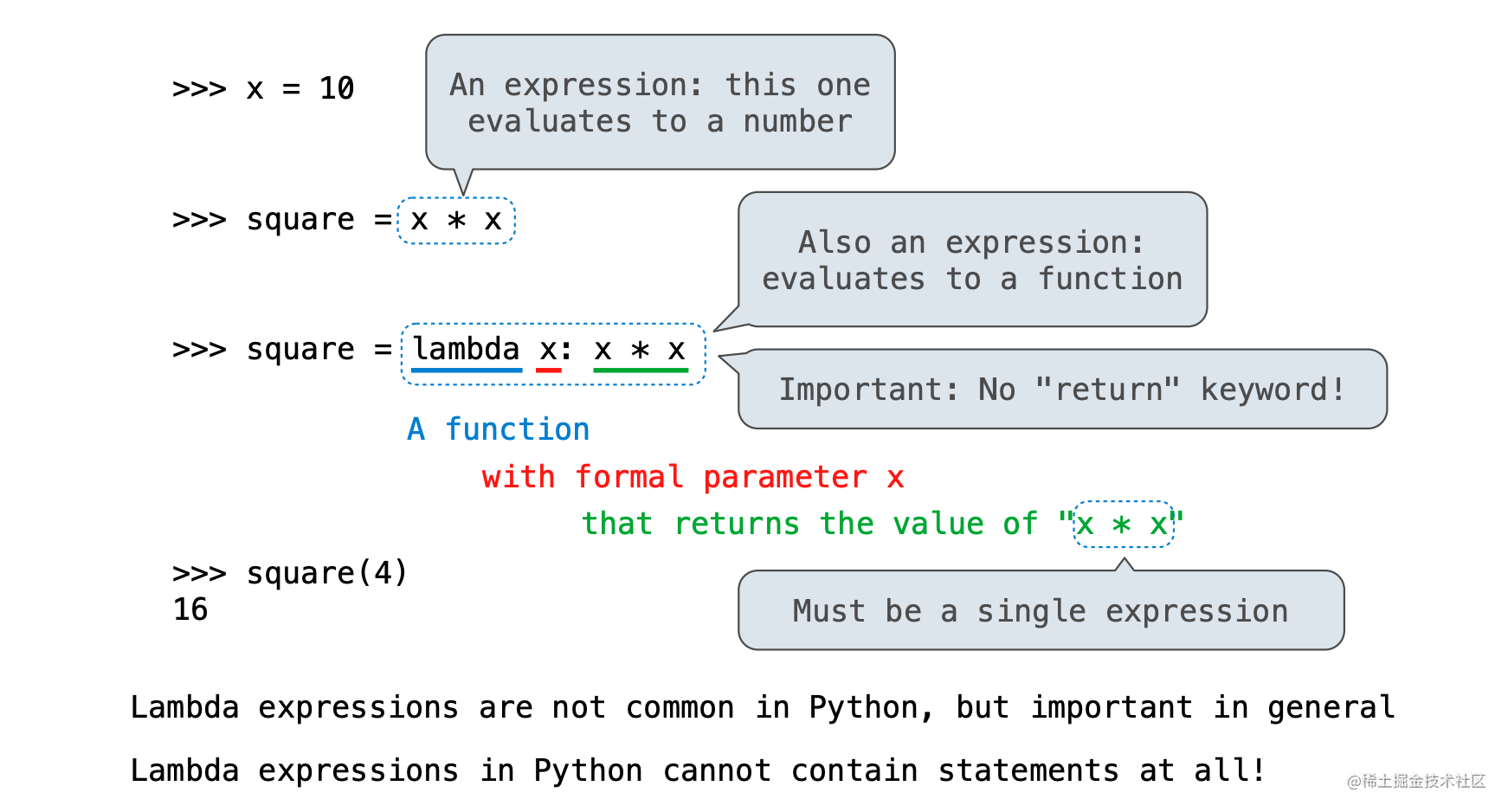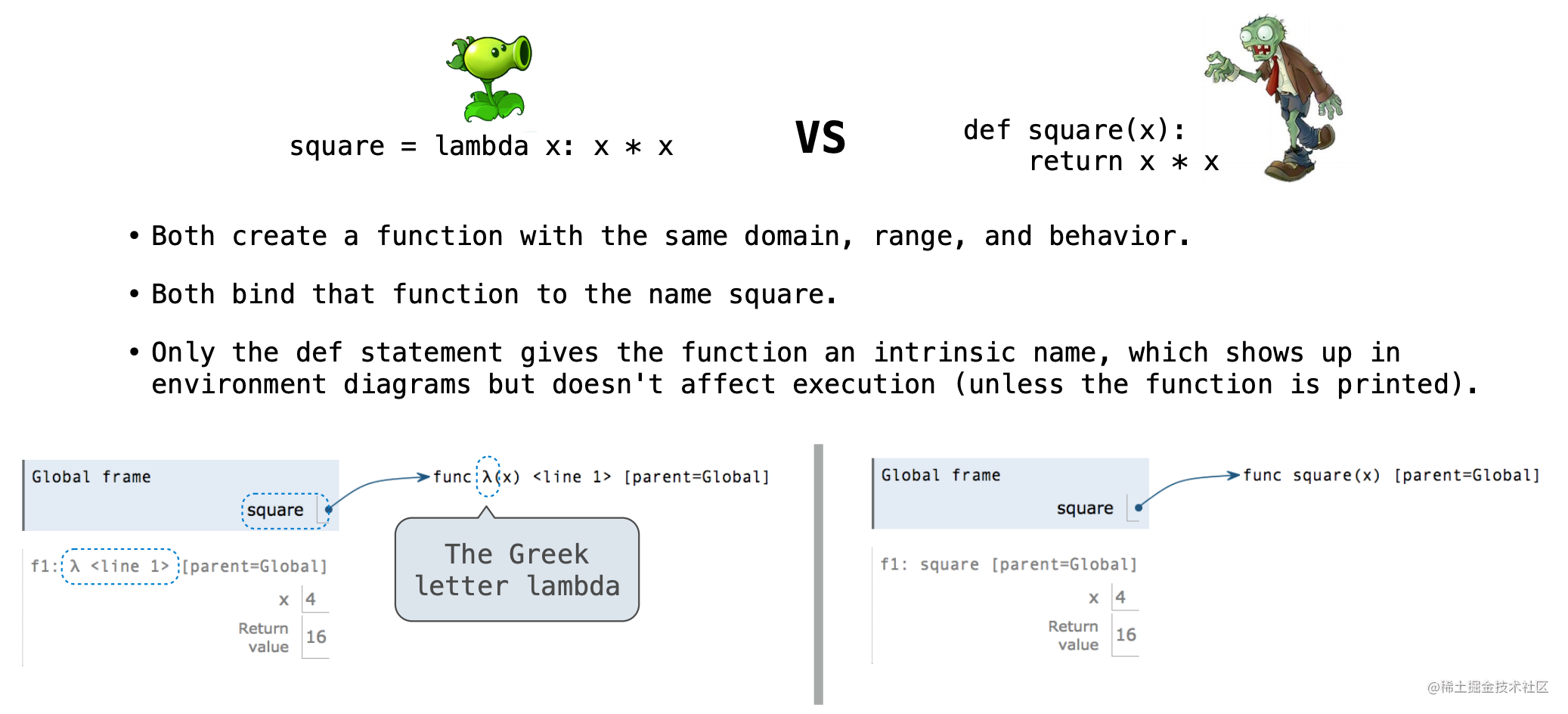# CS 61A: SICP - week2 - 2

1. Designing Functions 1.1 Designing Functions

`````` A function's domain is the set of all inputs it might possibly take as arguments.
A function's range is the set of output values it might possibly return.
A pure function's behavior is the relationship it creates between input and output.

复制代码``````

1.2. A Guide to Designing Function

``````    Give each function exactly one job, but make it apply to many related situations
Don’t repeat yourself (DRY): Implement a process just once, but execute it many times
Define functions generally

2. Designing Functions

1. Higher-Order Functions

3.1

``````    The common structure among functions may be a computational process, rather than a number

def cube(k): return pow(k, 3)
def summation(n, term):
total, k = 0, 1
while k <= n:
total, k = total + term(k), k + 1

3.2. Functions as Return Values

``````    Functions defined within other function bodies are bound to names in a local frame

def adder(k): return k + n

3.3 The purpose of higher-order functions

``````    Functions are first-class: functions can be manipulated as values in our programming language

hight-order function: a function that takes a function as an argument value or returns a function as a return value

higher-order functions:
. express general methods of computation
. remove repetition from programs
. separate concers among functions

4. Lambda Expressions4.1 Lambda Expressions Versus Def Statements1. Return 5.1 Return Statements

`````` A return statement completes the evaluation of a call expression and provides its value: f(x) for user-defined function f: switch to a new environment; execute f's body return statement within f: switch back to the previous environment; f(x) now has a value Only one return statement is ever executed while executing the body of a function
复制代码``````
2. Conditional Expressions

`````` <consequent> if <predicate> else <alternative>
复制代码``````

Evaluation rule:

``````1. Evaluate the <predicate> expression.
2. If it's a true value, the value of the whole expression is the value of the <consequent>.
3. Otherwise, the value of the whole expression is the value of the <alternative>.